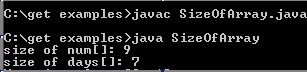# Get Array Size

In this example you will learn how to calculate length of the given array we have used length property. It returns the length of the given array.### Get Array Size

In this example you will learn how to calculate length of the given array we have used length property. It returns the length of the given array.# Get Array Size

To calculate length of the given array we have used length property. It returns the length of the given array.

In this tutorial we have declared two types array:

a) int array and

b) String array

There is the code to define the array in Java program:

int num[] = {1, 2, 3, 4, 5, 6, 7, 8, 9};
String days[] = {"Sunday","Monday","Tuesday", "Wednesday",
"Thursday","Friday","Saturday"};

We have used these two types of array and the explained you how to get the size of these array.?

The array.length is used to find out the size of the array.

The code array.length retuns the no of elements in the array.

Here is the video tutorial of "How to get size of array in Java?":

Here is the full code of the example code exlained in the video tutorial.

 ``` public class SizeOfArray {   public static void main (String args []) {     int num[] = {1, 2, 3, 4, 5, 6, 7, 8, 9};   String days[] = {"Sunday","Monday","Tuesday", "Wednesday",   "Thursday","Friday","Saturday"};   System.out.println("size of num[]: " + num.length);   System.out.println("size of days[]: " + days.length);   } }```

Output will be displayed as: